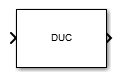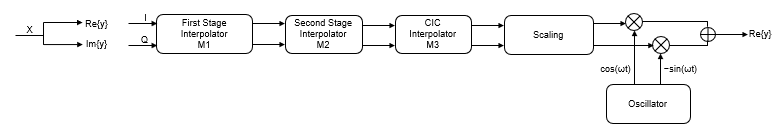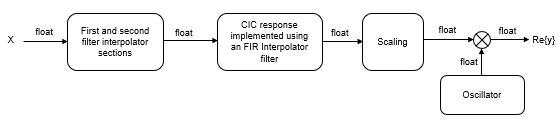# Digital Up-Converter

Interpolate and translate digital signal from baseband to intermediate frequency (IF) band

•Libraries:
DSP System Toolbox / Signal Operations

## Description

The Digital Up-Converter (DUC) block converts a complex digital baseband signal to a real passband signal.

The DUC block upsamples the input signal using a cascade of three interpolation filters. The block frequency upconverts the upsampled signal by multiplying it by the specified center frequency of the output signal. This block designs the interpolation filters according to the filter parameters you set in the block dialog box.

## Ports

### Input

expand all

Specify the input signal as a column vector of real or complex values.

When the input data type is `double` or `single` precision, the output data type is the same as that of the input. When the input data type is a signed integer or fixed-point, the output data type is defined by the Output parameter on the Data Types tab.

Data Types: `single` | `double` | `int8` | `int16` | `int32` | `int64` | `fixed point`
Complex Number Support: Yes

### Output

expand all

The block outputs the upconverted and upsampled signal as a column vector of real values. The length of the output is equal to the length of the input multiplied by the value that you specify in the Interpolation factor parameter.

When the input data type is `double` or `single` precision, the output data type is the same as that of the input. When the input data type is a signed integer or fixed-point, the output data type is defined by the Output parameter on the Data Types tab.

Data Types: `single` | `double` | `int8` | `int16` | `int32` | `int64` | `fixed point`

## Parameters

expand all

### Main Tab

Specify the interpolation factor as a positive integer > `1`, or as a 1-by-2 or 1-by-3 vector of positive integers.

When you set this parameter to a scalar value, the block applies the same interpolation factor to the three interpolation filtering stages.

When you set this parameter to a 1-by-2 vector, the block applies the second and third values in the vector to the second and third filtering stages, respectively, and bypasses the first filter stage. Both elements of the Interpolation factor must be greater than `1`.

When you set this parameter to a 1-by-3 vector, the block applies the values in the vector to the corresponding filtering stages. The second and third elements of Interpolation factor must be greater than `1`, and the first element must be `1` or `2`.

When you select this check box, the block designs filters with the minimum possible order that meets the requirements specified in these parameters:

• Passband ripple of cascade response (dB)

• Stopband attenuation of cascade response (dB)

• Two sided bandwidth of input signal (Hz)

• Source of stopband frequency

• Stopband frequency (Hz)

When you clear this check box, the block designs filters with orders that you specify in Order of first filter stage, Order of CIC compensation filter stage, and Number of sections of CIC interpolator. The filter designs meet the passband and stopband frequency specifications that you set in Two sided bandwidth of input signal (Hz), Source of stopband frequency, and Stopband frequency (Hz). By default, this check box is selected.

Specify the order of the first filter stage as an even positive integer. When you specify Interpolation factor as a 1-by-2 vector, the block ignores the value in this parameter because the block bypasses the first filter stage.

#### Dependencies

To enable this parameter, clear the Minimum order filter design parameter.

Specify the number of sections in the CIC interpolator as a positive integer.

#### Dependencies

This parameter appears when you clear the Minimum order filter design parameter.

Specify the source of the stopband frequency as `Auto` or `Property`.

When you set this parameter to `Auto`, the block places the cutoff frequency of the cascade filter response at approximately Fc = SampleRate/2 Hz, and computes the stopband frequency as Fstop = Fc + TW/2. SampleRate is computed as `1`/ Ts, where Ts is the sample time of the input signal. TW is the transition bandwidth of the cascade response, computed as 2×(FcFp), and the passband frequency, Fp, equals Bandwidth/2.

When you set this parameter to `Property`, specify the stopband frequency in the Stopband frequency (Hz) parameter.

Specify the oscillator type as one of the following:

• `Sine wave` (default) — The block performs frequency upconversion on the output of the interpolation filter cascade using a complex exponential signal obtained from samples of a sinusoidal trigonometric function.

• `NCO` — The block performs frequency upconversion with a complex exponential obtained using a numerically controlled oscillator (NCO).

Specify the center frequency of the output signal in Hz as a double-precision positive scalar. The value of this parameter must be less than or equal to half the product of the SampleRate times the total interpolation factor. SampleRate is computed as `1`/ Ts, where Ts is the sample time of the input signal. The block up converts the input signal so that the output spectrum centers at the frequency you specify in Center frequency of output signal (Hz).

Specify the order of the CIC compensation filter stage as a positive integer.

#### Dependencies

To enable this parameter, clear the Minimum order filter design parameter.

Specify the two-sided bandwidth of the input signal in Hz as a positive integer. The block sets the passband frequency of the cascade of filters to half of the value you specify in this parameter.

Specify the stopband frequency in Hz as a double-precision positive scalar.

#### Dependencies

To enable this parameter, set the Source of stopband frequency to `Property`.

Specify the passband ripple of the cascade response in dB as a double-precision positive scalar. When you select the Minimum order filter design parameter, the block designs the filters so that the cascade response meets the passband ripple that you specify in Passband ripple of cascade response (dB).

#### Dependencies

To enable this parameter, select the Minimum order filter design parameter.

Specify the stopband attenuation of the cascade response in dB as a double-precision positive scalar. When you select the Minimum order filter design parameter, the block designs the filters such that the cascade response meets the stopband attenuation that you specify in this parameter.

#### Dependencies

To enable this parameter, select the Minimum order filter design parameter.

Specify the number of NCO accumulator bits as an integer scalar in the range [`1 128`].

#### Dependencies

To enable this parameter, set Type of oscillator to `NCO`.

Specify the number of NCO quantized accumulator bits as an integer scalar in the range [`1 128`]. This value must be less than the value you specify in Number of NCO accumulator bits.

#### Dependencies

To enable this parameter, set Type of oscillator to `NCO`.

When you select this parameter, the block applies dither to the NCO signal according to the number of dither bits you specify in Number of NCO dither bits.

#### Dependencies

To enable this parameter, set Type of oscillator to `NCO`.

Specify the number of NCO dither bits as an integer scalar smaller than the number of accumulator bits in Number of NCO accumulator bits.

#### Dependencies

To enable this parameter, set Type of oscillator to `NCO` and select the Dither control for NCO parameter.

When you select this parameter, the block computes the sample rate as `N`/Ts, where N is the frame size of the input signal, and Ts is the sample time of the input signal. When you clear this parameter, the block sets the sample rate to the value in Input sample rate (Hz).

Specify the sample rate of the input signal in Hz as a positive scalar. The value of this parameter multiplied by the total interpolation factor must be greater than or equal to twice the value in Center frequency of output signal (Hz).

#### Dependencies

To enable this parameter, clear the Inherit sample rate from input parameter.

Click this button to open the Filter Visualization Tool (FVTool) and display the magnitude and phase response of each stage as well as the cascade of stages in the Digital Up-Converter. The response is based on the values you specify in the block parameters dialog box.. Changes made to these parameters update FVTool.To update the magnitude response while FVTool is running, modify the parameters in the dialog box and click .

Specify the type of simulation to run. You can set this parameter to:

• `Code generation` (default)

Simulate model using generated C code. The first time you run a simulation, Simulink® generates C code for the block. The C code is reused for subsequent simulations, as long as the model does not change. This option requires additional startup time but provides faster simulation speed than ```Interpreted execution```.

• `Interpreted execution`

Simulate model using the MATLAB®  interpreter. This option shortens startup time but has slower simulation speed than ```Code generation```.

### Data Types Tab

Specify the data type of the output in the first, second, and third filter stages. You can set this parameter to:

• `Inherit: Same as input` (default) — The block inherits the Stage output data type from the input signal.

• `fixdt([],16,0)` — The block uses the fixed-point data type with binary-point scaling. Specify the sign mode of this data type as `[]` or `true`.

• An expression that evaluates to a data type, for example, `numerictype([],16,15)`. Specify the sign mode of this data type as `[]` or `true`.

The block casts the data at the output of each filter stage according to the value you set in this parameter. For the CIC stage, the casting is done after the signal has been scaled by the normalization factor.

For help with setting the stage output parameter, you can click the buttonto display the data type assistant.

Specify the data type of the block output. You can set this parameter to:

• `Inherit: Same as input` (default) — The block Inherits the output datatype from the input.

• `fixdt([],16,0)` — The block uses the fixed-point data type with binary-point scaling. Specify the sign mode of this data type as `[]` or `true`.

• An expression that evaluates to a data type, for example, `numerictype([],16,15)`. Specify the sign mode of this data type as `[]` or `true`.

For help with setting the Output parameter, you can click the buttonto display the data type assistant..

Specify the minimum value of the block output. The default value is `[]` (unspecified). Simulink software uses this value to perform:

• Simulation range checking (see Specify Signal Ranges (Simulink))

• Automatic scaling of fixed-point data types

Specify the maximum value of the block output. The default value is `[]` (unspecified). Simulink software uses this value to perform:

• Simulation range checking (see Specify Signal Ranges (Simulink))

• Automatic scaling of fixed-point data types

Select this parameter to prevent the fixed-point tools from overriding the data types you specify in the block dialog box.

## Block Characteristics

 Data Types `double` | `fixed point` | `integer` | `single` Direct Feedthrough `no` Multidimensional Signals `no` Variable-Size Signals `no` Zero-Crossing Detection `no`

expand all

## Algorithms

The digital up converter upsamples the input signal using a cascade of three interpolation filters. This algorithm frequency-upconverts the upsampled signal by multiplying it with a complex exponential that has the specified center frequency. In this case, the filter cascade consists of an FIR interpolation stage, a second stage for CIC compensation, and a CIC interpolator. The block diagram shows the architecture of the digital up converter.The scaling section normalizes the CIC gain and the oscillator power. It can also contain a correction factor to achieve the desired ripple specification. Depending on how you set the interpolation factor, the block bypasses the first filter stage. When the input data type is floating point, the algorithm implements an N-section CIC interpolation filter as a FIR filter with a response that corresponds to a cascade of N boxcar filters. The algorithm emulates a CIC filter with an FIR filter so that you can run simulations with floating-point data. When the input data type is a fixed-point type, the algorithm implements a true CIC filter with actual comb and integrator sections.

This block diagram represents the DUC arithmetic with floating-point inputs.For details about fixed-point operation, see Fixed Point.

## Version History

Introduced in R2015a